Ex 16.1

Chapter 16 Class 8 Playing with Numbers
Serial order wise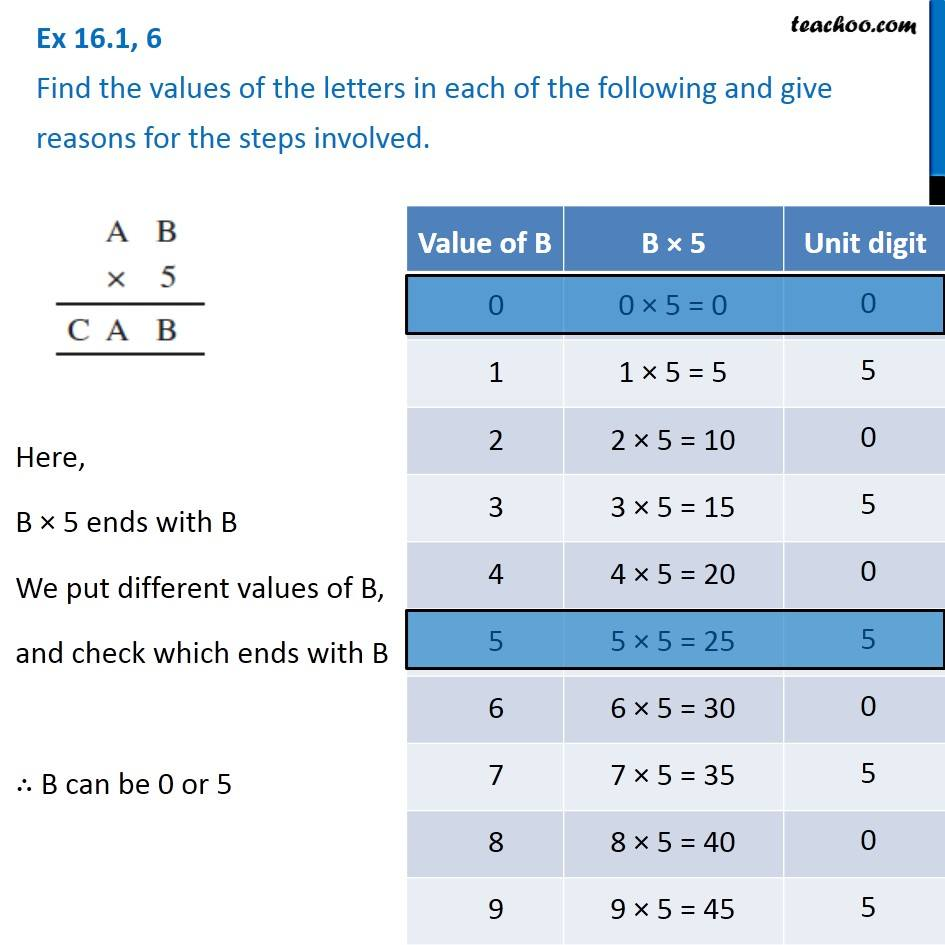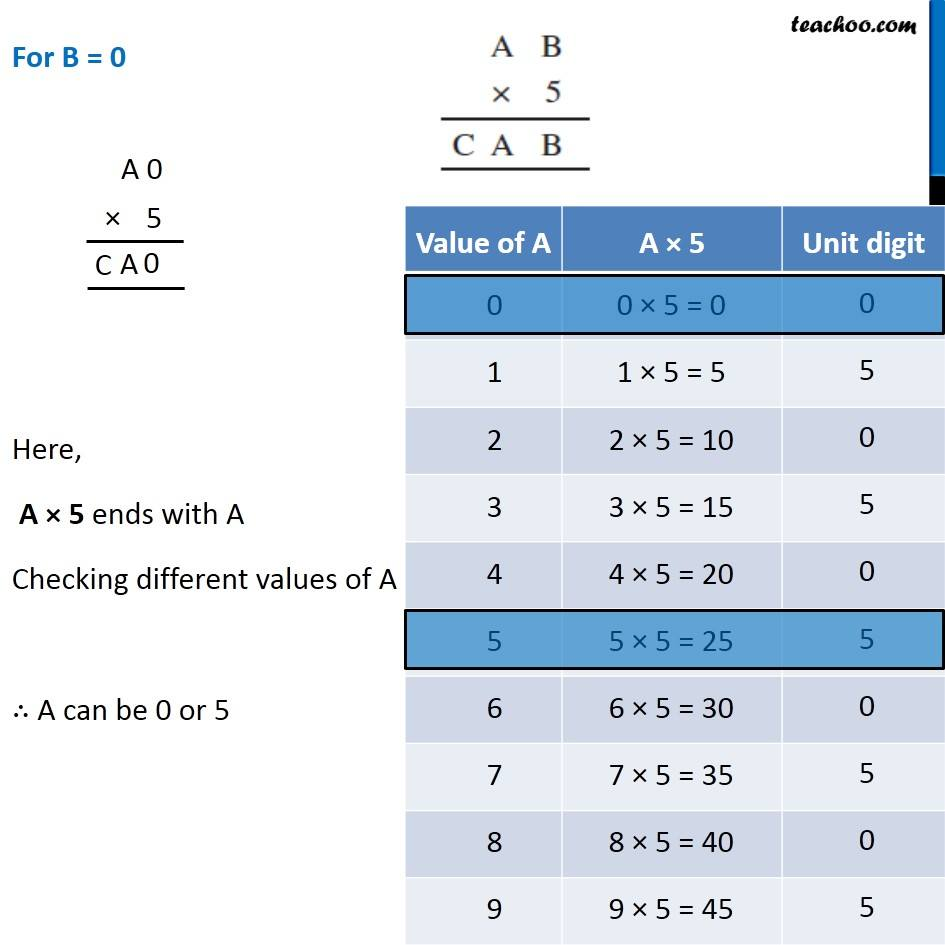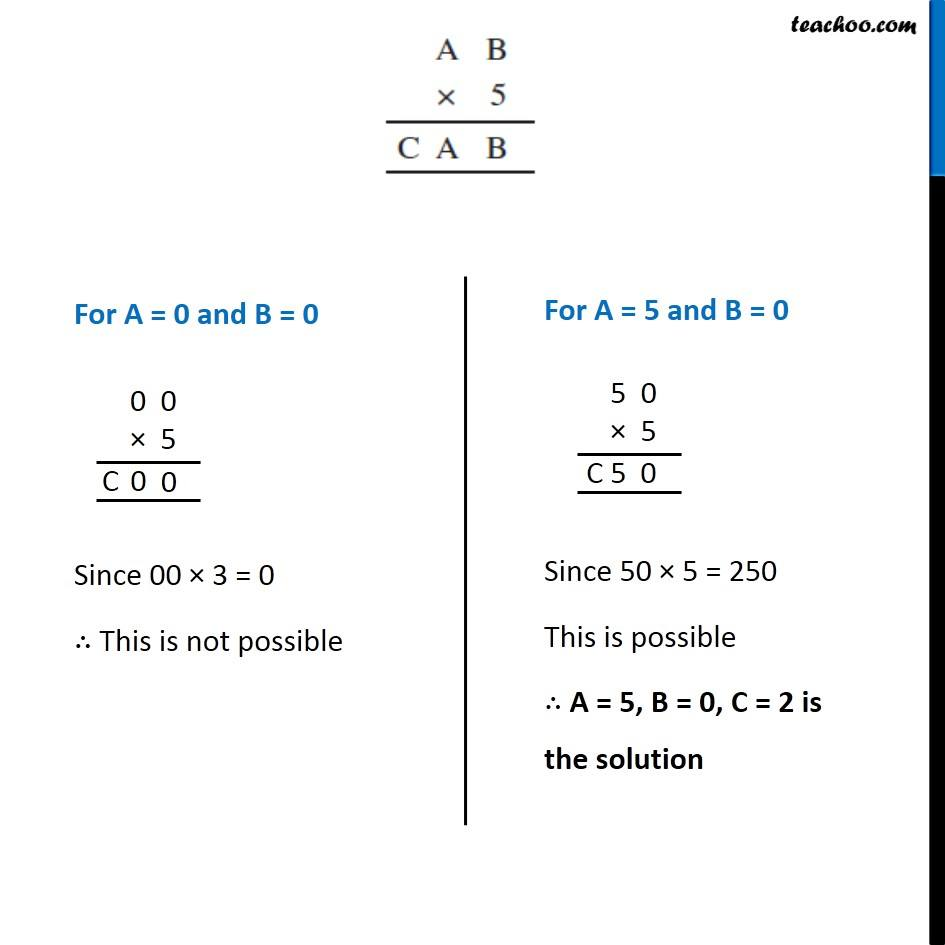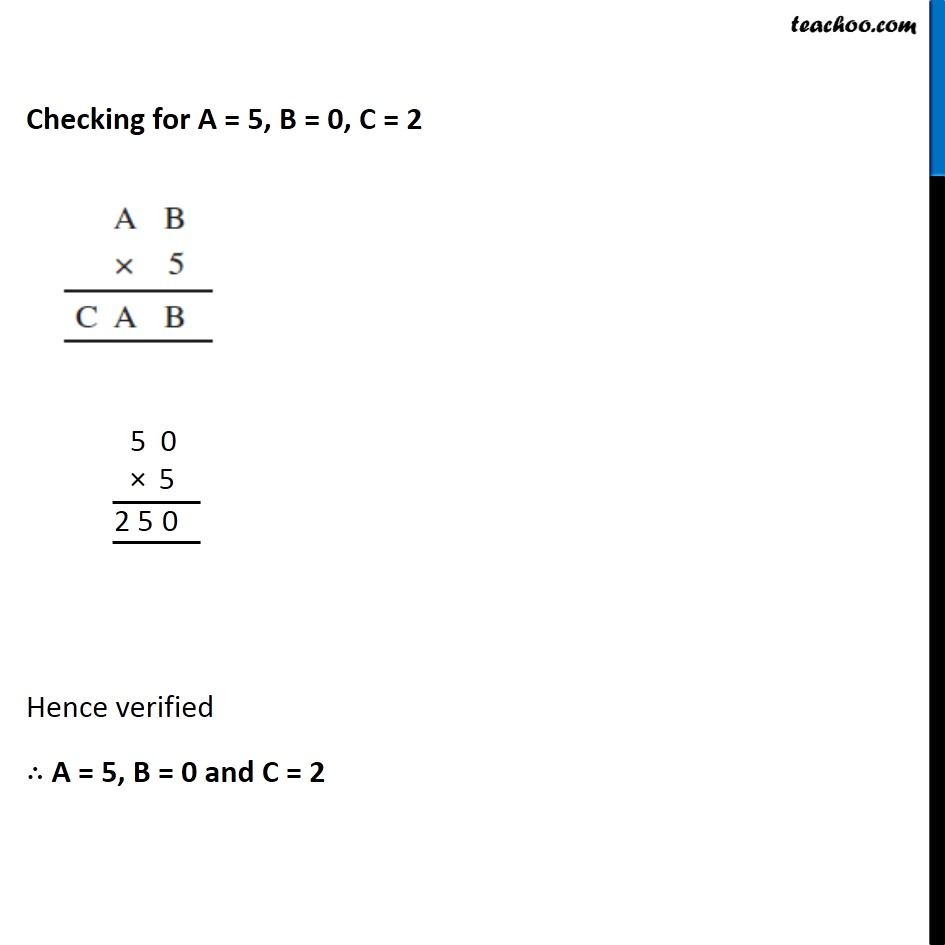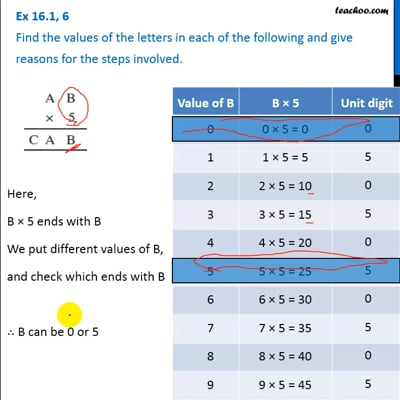This video is only available for Teachoo black users

Introducing your new favourite teacher - Teachoo Black, at only ₹83 per month

### Transcript

Ex 16.1, 6 Find the values of the letters in each of the following and give reasons for the steps involved. Here, B × 5 ends with B We put different values of B, and check which ends with B ∴ B can be 0 or 5 For B = 0 Here, A × 5 ends with A Checking different values of A ∴ A can be 0 or 5 For A = 0 and B = 0 Since 00 × 3 = 0 ∴ This is not possible For A = 5 and B = 0 Since 50 × 5 = 250 This is possible ∴ A = 5, B = 0, C = 2 is the solution Checking for A = 5, B = 0, C = 2 Hence verified ∴ A = 5, B = 0 and C = 2